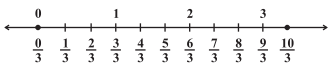# Worksheet on Representation of Rational Numbers on a Number Line | Plotting Rational Numbers on a Number Line Worksheet

Have you ever felt the topic Representation of Rational Numbers on a Number Line difficult? Not anymore! with our Worksheet on Representation of Rational Numbers on a Number Line and you can use them during your practice sessions. Clear all your queries by going through the solved examples on how to Plot Rational Numbers on a Number Line. For complete guidance on the Chapter Rational Numbers, you can use our Rational Numbers Worksheets.

The Questions covered in Placing Rational Numbers on the Number Line Worksheet include representing both positive and negative rational numbers on the number line. In general, any rational number is represented on the number line by a point. However, Positive Rational Numbers lie to the right of 0 and Negative Rational Numbers lie to the left of 0.

1. Between which two numbers does the Rational Number 8/3 lie?

Solution:

Given Rational Number is an Improper Fraction

Firstly change it to Mixed Fraction i.e. 2 2/3

The Whole Number is 2 therefore the rational number 8/3 lies between 2 and 3.

2. Between which two numbers the Rational Number -11/3 lie?

Solution:

Given Rational Number is an Improper Fraction

Firstly change it to Mixed Fraction i.e. -3 2/3

Therefore the given rational number lies between -3 and -4.

3. Which of the following statements is true or false

(i) -1/5 lies to the left of 0 on the number line.

(ii) The Rational Number 19/23 lies to the left of 0 on the number line.

(iii) -12/5 lies to the right of 0 on the number line.

(iv) The rational numbers 1/4 and -5/2 are on the opposite sides of 0 on the number line.

(v) The rational numbers (-11)/(-5) and (-4)/17 are on the opposite sides of 0 on the number line.

Solution:

(i) True

(ii) False

(iii) False

(iv) True

(v) True

4. Represent 4/3 on the Number Line?

Solution:

a) Firstly draw a number lineb) since the number is positive it will lie on the right side of 0. Since 4/3 can be written as mixed fraction 1 1/3 the number lie between 1 and 2.

c) Therefore, to the right side of zero mark 1/3, 2/3, 3/3, 4/3, 5/3, 6/3, and so on.5. Draw a Number Line and Represent the Following Positive Rational Numbers on the Number Line?

(i) 5/3

(ii) 1/3

(iii) 2/4

(iv) 3/7

(vi) 7/8

6. Represent each of the Negative Rational Numbers on the Number Line?

(i) (-5)/8

(ii) (-3)/14

(iii) (-2)/3

(iv) -3/4

(v) (-7)/3

(vi) 22/(-6)

(vii) (-13)/3